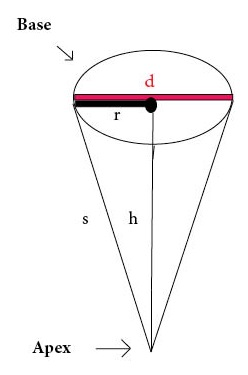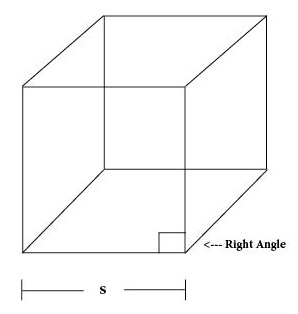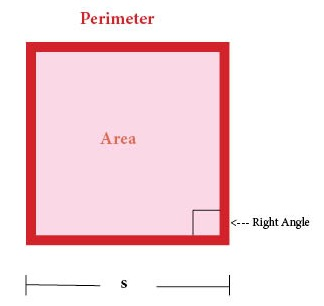# Parallelogram

Parallelogram = Flat Four-Sided Shape in which Opposite Sides are Parallel

# Trapezoid

Trapezoid = Flat Four-Sided Shape with Two Parallel Sides

# Cylinder

Cylinder = Three-Dimensional Shape with Circular Top and Base# Right Rectangular Prism

Right Rectangular Prism = Three-Dimensional Shape in which Opposite Faces are Equal (with all right angles)# Pyramid

Pyramid = Three-Dimensional Shape with Polygonal Base Connecting to a Point# Sphere

Sphere = 3-Dimensional Round Shape with All Surface Points Equidistant from Center# Cone

Cone = Three-Dimensional Shape with Circular Base Connecting to a Point# Triangle

Triangle = Flat Shape with Three Sides# Rectangle

Rectangle = Flat Shape with Four Sides (with four right angles)

# Cube

Cube = Three-Dimensional Shape with Six Square Faces (with all right angles)# Square

Square = Flat Shape with Four Equal Sides (with four right angles)